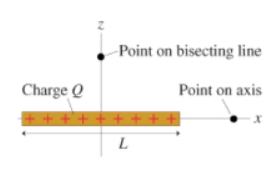# Problem: The figure showed a thin rod of length L and charge Q (Figure 1).Find an expression for the electric potential a distance z away from the center of rod on the line that bisects the rod. Give your answer in terms of L, Q, z, and appropriate constants.

###### FREE Expert Solution

The electric potential  is expressed as:

$\overline{){\mathbf{V}}{\mathbf{=}}\frac{\mathbf{k}\mathbf{Q}}{\mathbf{z}}}$

79% (217 ratings)###### Problem Details

The figure showed a thin rod of length L and charge Q (Figure 1).Find an expression for the electric potential a distance z away from the center of rod on the line that bisects the rod. Give your answer in terms of L, Q, z, and appropriate constants.